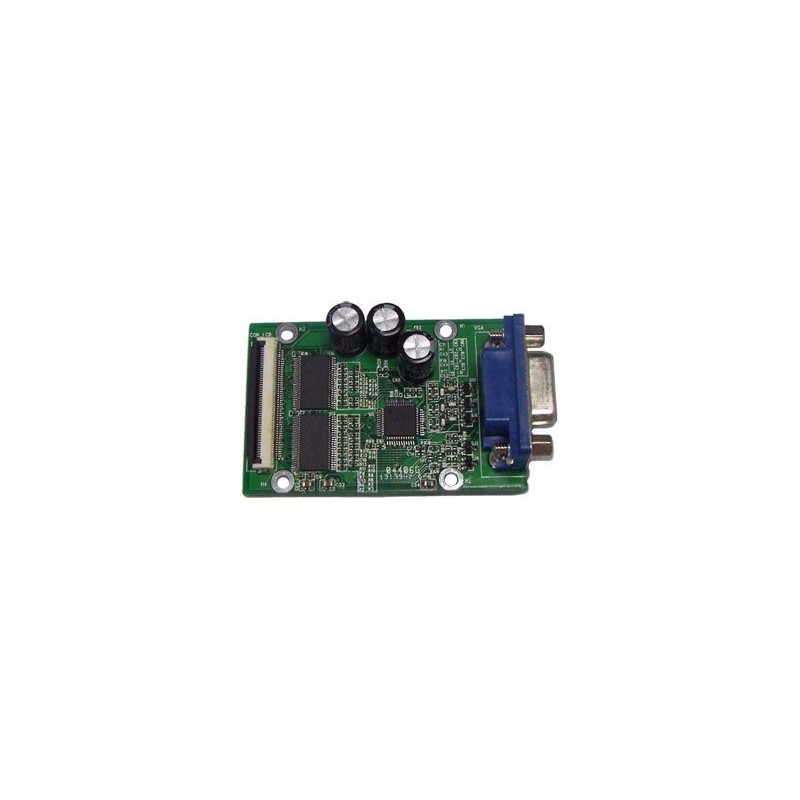﻿
Obniżka!Zobacz większe

# Handbook of Differential Equations: Evolutionary Equations

C.M. Dafermos, Eduard Feireisl

ID: 172654

### Więcej informacji

The aim of this Handbook is to acquaint the reader with the current status of the theory of evolutionary partial differential equations, and with some of its applications. Evolutionary partial differential equations made their first appearance in the 18th century, in the endeavor to understand the motion of fluids and other continuous media. The active research effort over the span of two centuries, combined with the wide variety of physical phenomena that had to be explained, has resulted in an enormous body of literature. Any attempt to produce a comprehensive survey would be futile. The aim here is to collect review articles, written by leading experts, which will highlight the present and expected future directions of development of the field. The emphasis will be on nonlinear equations, which pose the most challenging problems today.

. Volume I of this Handbook does focus on the abstract theory of evolutionary equations.
. Volume 2 considers more concrete problems relating to specific applications.
. Together they provide a panorama of this amazingly complex and rapidly developing branch of mathematics.

Contents
Preface
1. Euler Equations and Related Hyperbolic Conservations Laws, (G.-Q. Chen).
2. Blow-up of Solutions of Supercritical Parabolic Equations, (M. Fila).
3. The Boltzmann Equation and Its Hydrodynamic limits, (F. Golse).
4. Long-Time Behaviour of Solutions to Hyperbolic Equations with Hysteresis, (P. Krejci).
5. Mathematical Issues Concerning the Navier-Stokes Equations and some of their Generalizations, (J. Málek, K.R. Rajagopal).
6. Evolution of Rate-Independent Systems, (A. Mielke).
7. On the Global Weak Solutions to a Variational Wave Equation, (P. Zhang, Y. Zheng).